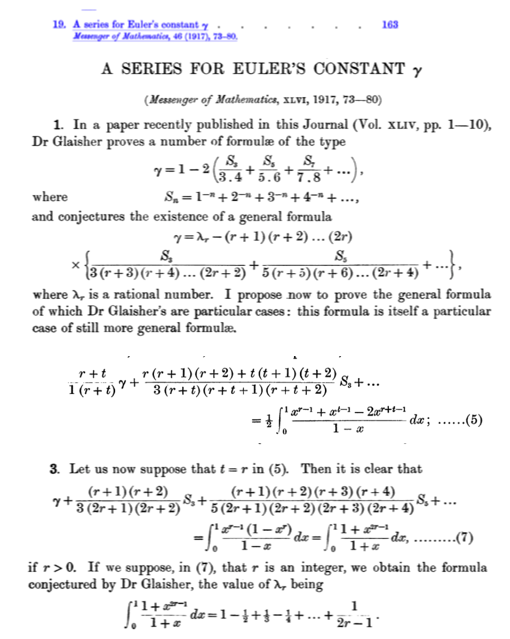# What is the collection of series that amount to $\gamma$ deduced by Ramanujan?

On p. 20 of an article by Borwein et al., it is stated that Ramanujan could generalize the following formula due to Glaisher $$\gamma = 2 - 2\log2 -2\sum_{n=3, \text{ odd}} \frac{\zeta(n)-1}{n(n+1)}$$ to infinitely many formulae for $$\gamma$$. They refer to Section 19 of Ramanujan's collected papers (which I don't have in my possession).

I wonder what this collection of series representations for $$\gamma$$ is, and whether it can be found in another (open-source) reference -- preferably including a derivation.

• The R.H.S transforms to $$1-2{\sum_{k=1} \frac{1}{k^3}(\frac{1}{3.4}+\frac{1}{5.6}\frac{1}{k^2}+\frac{1}{7.8}\frac{1}{k^4}+.....)}$$. And this inside sum will be $kf(\frac{1}{k} ,k>1$ where $f^{(2)}(x)=\frac{1}{1-x} ,|x|<1$. – Alapan Das May 18 at 11:25

Here is a scan from Ramanujan's 1917 paper on the generalized $$\gamma$$ formulas (equations 5 and 7). The reference in Messenger of Mathematics is a journal that no longer exists. Only the volumes through 1901 are freely accessible online.